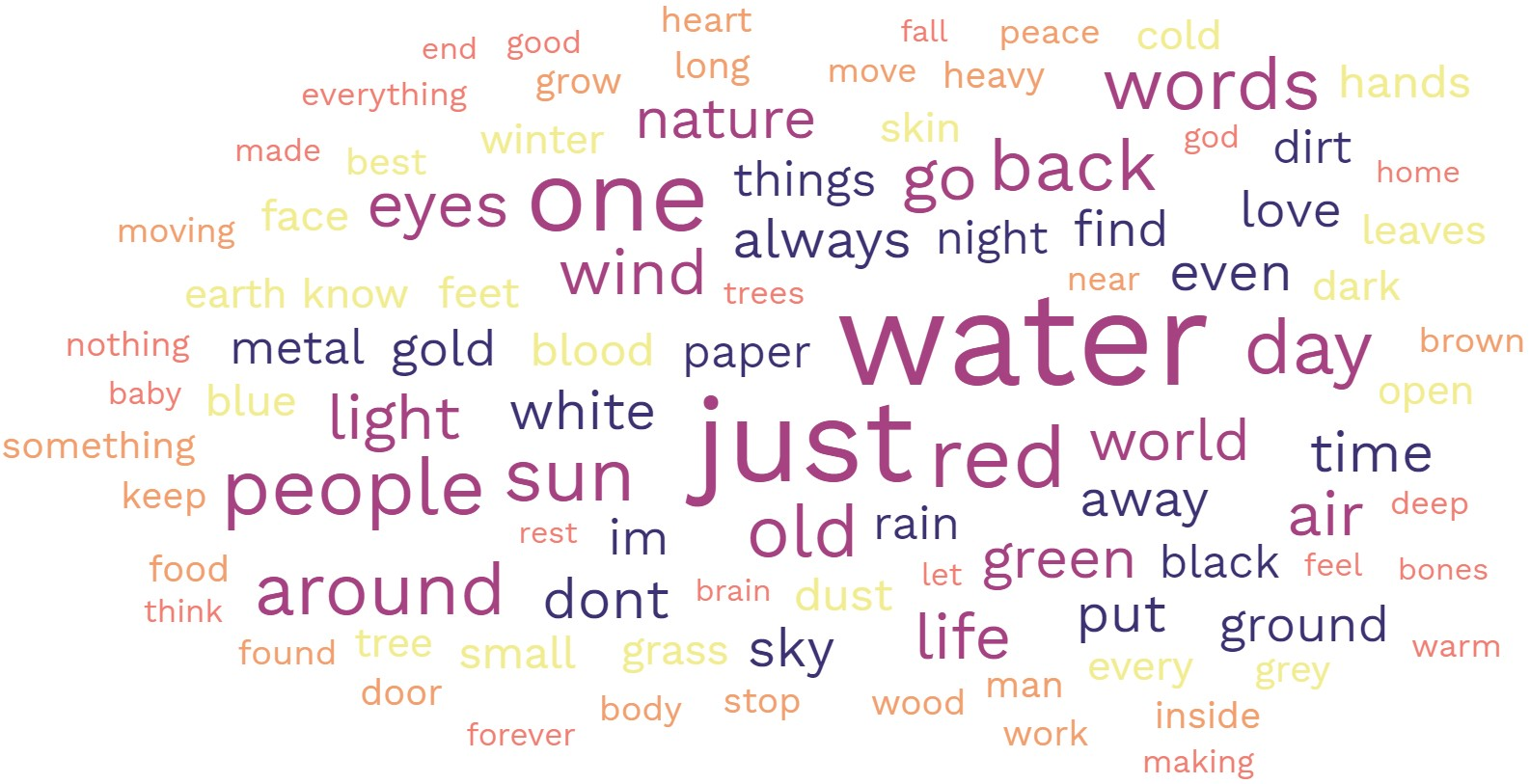# Number Haiku

7/1/19

In this article I will talk about making a haiku using the numbers 0-9 (ie. 0, 1, 2, ..., 9). I will denote this set of numbers as S.

Why would someone want to make a haiku like this? Good question. Maybe they like haikus or alternative poetry? Maybe they like numbers? Perhaps they are making a code? Or, maybe they just like the challenge of it. For me, I like the challenge, like numbers, and, probably like most people, find certain numbers significant in my life. Consider, for example, 911, 711 (I like Slurpees, OK?), 007, 411, 24/7, 777, 666, ages, addresses, phone numbers, birth, death, anniversary dates, text converted to numbers, and many more possibilities.

All of the numbers in S have one syllable, except 0 and 7 which have 2. Therefore, we can construct 5 and 7 syllable lines pretty easily by concatenating the numbers from S.

I put the numbers from S in a column and randomly sample from them to fill the 5, 7, and 5 lines in the haiku. I keep track of the cumulative syllable count for each line. I don't allow the counts to get over 5, 7, or 5, respectively. Just to be clear here, I am not saying a haiku must always be 575 or that 575 is the definition of a haiku.

Because of this constraint, what can happen is that lines can have one syllable less than 5, 7, or 5. For example, say the first line only has a syllable count of 4 because the next number would push the syllable count over 5 (for example if 0 were to be selected). I simulated thousands of haikus and found out that this scenario occurs about 42% of the time.

To remedy this, when this happens I simply randomly select from the list S1, where S1 is a list of all numbers that are one syllable (ie. the numbers in S but without the numbers 0 or 7), and concatenate this number on the end (although it could be inserted anywhere in a line). Doing this I automatically get 5, 7, and 5 lines with the correct syllable counts.

I also make two versions of each haiku; the word version and the number version. For example, the two versions for a 5 line from a haiku might be "six four zero two" or "6402".

Here are some examples:

```zero eight nine three
four four nine one five nine six
zero six six six
```

```zero four three eight
seven seven seven eight
seven five six five
```

Here are their numerical versions

```0893
4491596
0666
```

```0438
7778
7565
```

I also made a tab in the spreadsheet that just creates binary ones. That is, S = {0,1}. And just for giggles, I also convert these binary 575 lines to their decimal equivalent. Here is an example of a binary number haiku:

```one zero one one
one one zero zero one
one one one one one
```

And the numerical version

```1011
11001
11111
```

One could also use this general idea to mix words with numbers in a haiku, or randomly sample from any words you want with any constraint you want.

Here is a spreadsheet that creates these number haikus. You are free to edit the spreadsheet, but please credit Statisticool.com if you use it.